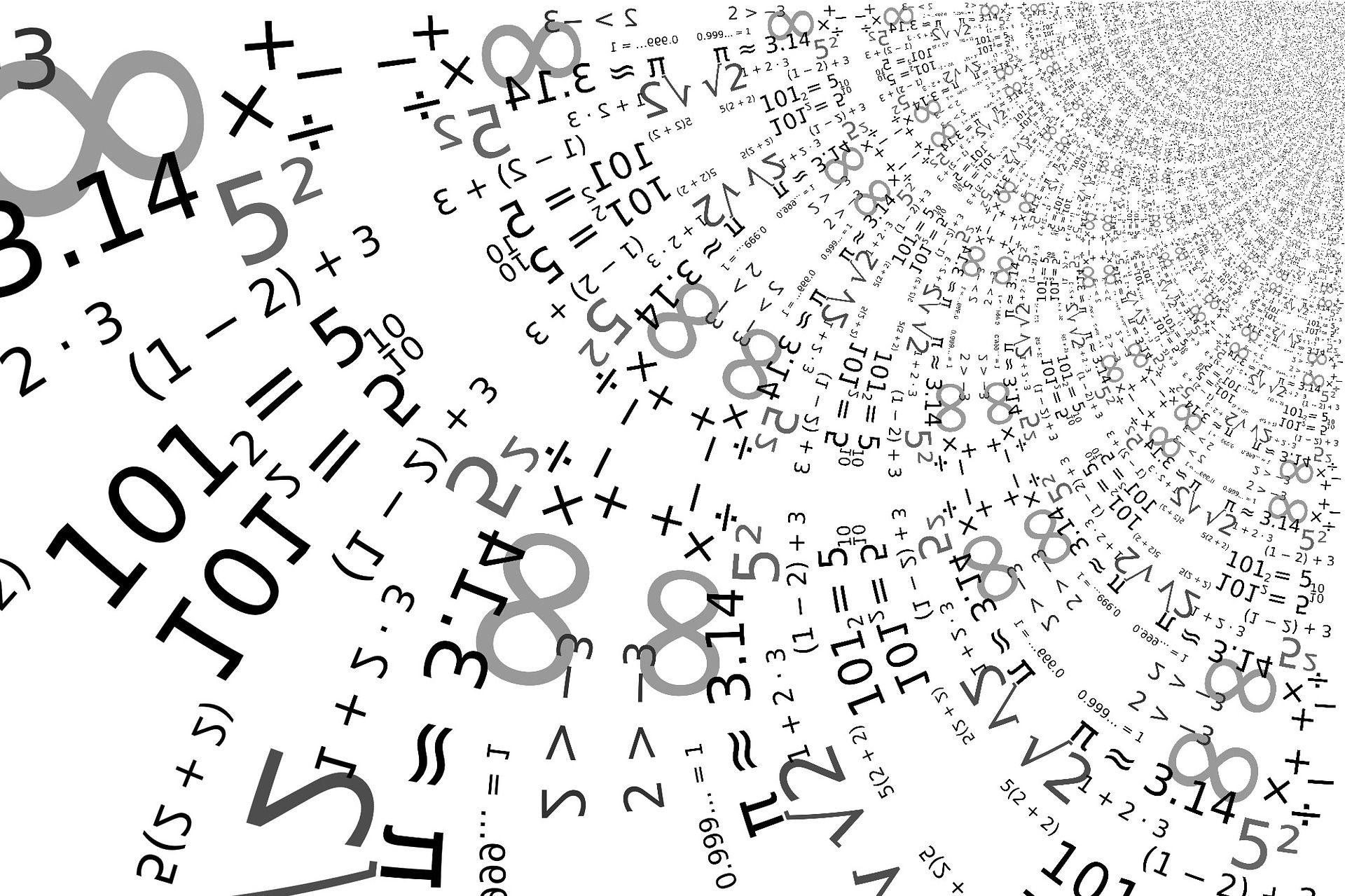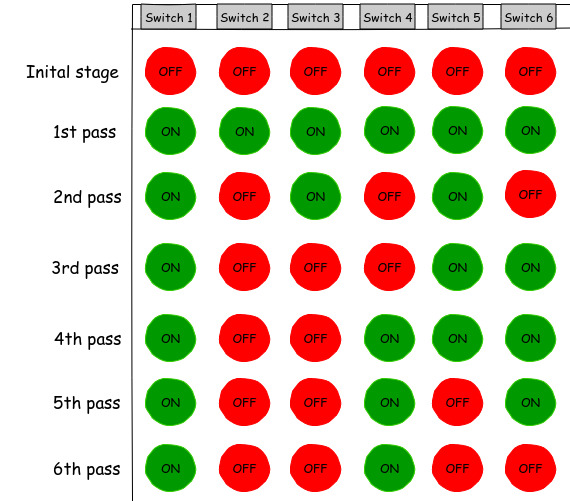# Decreasing complexity one step at a time“Knowing it and seeing it are two different things.” ― Suzanne Collins

I solve a lot of competitive programming(cp) problems in my free time. Recently I got a very interesting problem. I discovered many ways of solving it. Every new solution I got was better than the previous one. This blog post is just about showing that what we see is not always the reality.

## The problem

This is the problem I encountered - Light, more light

Let me explain the question first in a concise manner. There are N toggle switches (i.e. if pressed, then it will turn on. Another press will turn it off) arranged linearly. There are N switches, so we have to go N times through all the switches. Assume the term ‘i’ every time we go. In every ith term, we have to press the switch(es) which all are divisible by i. After repeating the process N times, in the end, we have to tell that the last switch is ON or OFF.

### Example

For example, assume N = 6 means there are 6 switches as of now. Initially, all the switches are set to OFF.

• For the first pass, i = 1 and every number from 1 to 6 is divisible by 1 so all switches get pressed.
• For the second pass, i = 2. Only 2, 4 and 6 are divisible by 2 so switch 2, 4 and 6 are pressed and change their state.
• For the third pass, i = 3. This time 3 and 6 are divisible by 3 so they get pressed and change their state.

and so on…(see picture)After simulating the whole process the last switch (i.e. switch 6) is OFF. So the result will be OFF.

## Solutions

There are many ways of solving it. Let’s see.

### * The First Glance *

The very first solution which came into my mind was to do the simulation.

Algorithm:

• Get N from the user.
• Create an array of N+1 elements all set to 0.
• Loop through 1 to N.
• Check all the element if they are divisible by i then change it from 1 to 0 or 0 to 1.
• After loop gets over, print “ON” if A[n] is 1 else print “OFF”.

Pseudocode

``````PROGRAM slow_code:
DECLARE A[n+1] = {0}
FOR i IN 1 TO n: DO
j = i
WHILE j <= n:
IF A[j] == 1:
A[j] = 0
ELSE
A[j] = 1
ENDIF;
j = j + i
ENDWHILE;
ENDFOR;

IF A[n] == 1:
WRITE "ON"
ELSE:
WRITE "OFF"
ENDIF;
ENDPROGRAM
``````

Time complexity   - O(nlogn)
Space complexity - O(n)

### * The Elimination Round *

When you don’t know what you want, first figure out what you don’t want. - Biswa, Laakhon Mein Ek

If you see the problem very keenly, then you can notice that we don’t need to know about the rest of the switches except the last one. It means, we only need to know the numbers between 1 to N which can divide N.

Algorithm:

• Get N from the user.
• Set switch to False.
• Loop through 1 to N.
• For every i, if N is divisible by i then change switch from False to True or True to False.
• After loop gets over if the switch is set to True print “ON” else print “OFF”.

Pseudocode

``````PROGRAM fast_code:
switch = False
FOR i IN 1 TO n: DO
IF n%i == 0:
IF switch == False:
switch = True
ELSE:
switch = False
ENDIF;
ENDIF;
ENDFOR;
IF switch == True:
WRITE "ON"
ELSE:
WRITE "OFF"
ENDIF;
ENDPROGRAM
``````

Time complexity   - O(n)
Space complexity - O(1)

### * The Ninja Way *

Now it’s time to be the ninja. From the previous solution, we can observe a very important thing. What we want to know is the count of numbers that can divide N is even or odd. If the count is even, it will set the switch to False and if it’s odd that means it will set it to True.E.g. For number 12, Initally the switch is set to OFF.

Due to 1, it will change it to ON.
Due to 2, it will change it to OFF.
Due to 3, it will change it to ON.
Due to 4, it will change it to OFF.
Due to 6, it will change it to ON.
Due to 12, it will change it to OFF.

So all we want to know now is that the factors count of N is even or odd. If we get any way to know that then we can easily get the answer. This answer is hidden inside a mathematical concept “Perfect Square”.

#### Perfect Square

def : A perfect square is a number that can be expressed as the product of two equal integers. e.g. 1(1x1), 4(2x2), 16(4x4), 25(5x5), etc.

There is one property of perfect square. The factor count of perfect square is always odd. The factors count of rest of the numbers is always even. The question is HOW??

If you take any number X, you can write it as a product of two numbers. There can be multiple ways. See the picture below to understand the differece between a perfect square and a non-perfect square.In the above example, first see the right hand side. For number 12, the first three lines are same as the last three lines. Each lines are written as a * b, where a is not equal to b in all the lines. i.e. the count will always be even because the factors come in pairs.

Now let’s see the left hand side. For number 16, the first two lines are same as last two lines. These four lines is also written as a * b, where a is not equal to b. The special part is the third line. It is written as a * b but here a is equal to b. i.e. the first and last two lines makes the factor count even but due to third line, it will become odd because the third line gives only one factor.

PHEW!

So, to know that the switch is ON or OFF all we really need to know is that the number given is perfect square or not. If it is perfect square, then the switch will be ON else it will be OFF.

Pseudocode

``````PROGRAM fastest_code:
y = FLOOR(SQRT(n))
IF y*y == n:
WRITE "ON"
ELSE:
WRITE "OFF"
ENDIF;
ENDPROGRAM
``````

Python implementation

``````import math
switch_status = lambda x:"ON" if math.floor(math.sqrt(x))**2==x else "OFF"
print(switch_status(int(input())))
``````

Time complexity   - Depends on the sqrt function. know more
Space complexity - O(1)

## Conclusion

Coding is not the toughest part, thinking is. At the very beginning, we tried to simulate the whole process to get the result. Then we removed the noise to make the problem less lumbering. We end up checking that the number is a perfect square or not. Can you see the beauty of thoughts that are deeply rooted in this whole journey? To know the answer that lies within, we first need to find out what is the real question. This time, it was just asking to know that the number is a perfect square or not but the question in itself was beautifully crafted to make it sound very complex. It all boils down to this quote -

The art of programming is the art of organizing complexity, of mastering multitude and avoiding its bastard chaos as effectively as possible. - E. W. DIJKSTRA